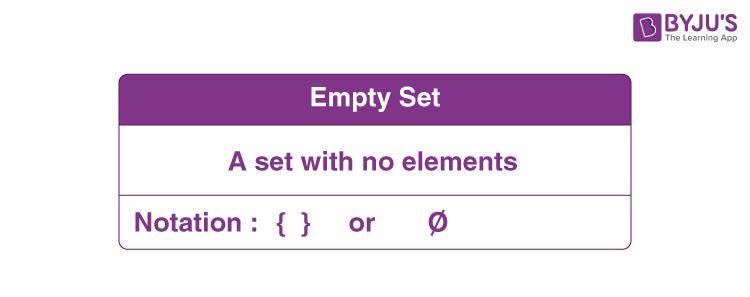Jet Set Go! All about Aeroplanes Jet Set Go! All about Aeroplanes

# Empty Set

In mathematics, a set is a well-defined collection of objects or elements. Based on the number of elements in a set, we can define finite and infinite sets. However, we have another particular type of set called the empty set. The empty set is the unique set having no elements such that its cardinality is 0. The empty set is referred to as the “null set” in most textbooks and publications. Unfortunately, though, the null set is a different notion within the context of measure theory. It represents a set of measure zero that means this set is not indeed empty. Consequently, the empty set may also be called the void set. In this article, you will learn the mathematical definition of empty set, symbol, examples and properties in detail.

## Empty Set Definition

A set which does not contain any element is called the empty set or the null set or the void set. For example, the set of the number of outcomes for getting a number greater than 6 when rolling a die. As we know, the outcomes of rolling a die are 1, 2, 3, 4, 5, and 6. Thus, the set with numbers greater than 6 here will be { }. That means there will be no elements and is called the empty set.

## Empty Set Symbol

The empty set is denoted by the symbol “Φ” and “φ” or { }.## Empty Set Examples

Let’s have a look at a few examples of empty sets given below.

(i) Consider set A = {x : 3 < x < 4, x is a whole number} and this set A is the empty set, since there is no whole number between 3 and 4.

(ii) Suppose B = {x: x is a composite number less than 4} such that B is the empty set since 4 is the smallest composite number.

(iii) The set C = {x : x2 – 3 = 0 and x is rational number} and it is the empty set since the equation x2 – 3 = 0 is not satisfied by any rational value of x.

(iv) The set D = { x : x3 = 27, x is even} is the empty set, since the equation x3 = 27 is not satisfied by any even value of x.

### Properties of Empty Set

The most important properties of an empty set are listed below:

Cardinality: The number of elements of the empty set or the cardinality of empty set is zero, |φ| = 0

Empty set is a subset of every set: For any set A, the empty set is a subset of A, i.e. φ ⊆ A; ∀ A

Empty set subset: The only subset of an empty set is the empty set itself, i.e. A ⊆ φ ⇒ A = φ

Cartesian product with empty set: The Cartesian product of A and the empty set is the empty set, i.e. A × φ = φ; ∀ A

Power set of empty set: The power set of the empty set is the set containing only the empty set, i.e. 2φ = {φ}

Union with Empty set: The union of A with the empty set is the set A itself, i.e. A ⋃ φ = A; ∀ A

Intersection with Empty: The intersection of A with the empty set is again the empty set, i.e. A ⋂ φ = φ; ∀ A

### Empty Set is Finite or Infinite

An empty set is a finite set since its cardinality is defined and is equal to 0. As we know, a set is said to be infinite if the number of elements in it are infinite, i.e. its cardinality is ∞ or not defined, whereas a finite set contains a countable number of elements.

## Video Lesson on What are Sets## Frequently Asked Questions on Empty Set

### What is an empty set example?

The set of natural numbers less than 1 is an empty set since the natural numbers start from 1, and hence, there will be no elements in the required set.

### What is Ø in math?

In maths, the symbol Ø is used in set theory to represent the empty set.

### What is a null or empty set?

A set which does not contain any element is called the empty set or the null set or the void set.

### How do you write an empty set?

A set with no elements is called an empty set and it can be written as { }.

### Can an empty set be a proper subset?

The empty set is a proper subset of every set except for the empty set and no set is a proper subset of itself.

### Is Empty set countable?

Yes, the empty set is countable. However, the cardinality of an empty set is 0.